EPL 84 19001 (5pp)
doi:10.1209/0295-5075/84/19001

Polarization of superluminal γ-rays: Tachyonic flare spectra of quasar 3C 279

R. Tomaschitz

Department of Physics, Hiroshima University - 1-3-1 Kagami-yama, Higashi-Hiroshima 739-8526, Japan

E-mail: tom@geminga.org

Received 20 August 2008, accepted for publication 29 August 2008
Published 17 September 2008

 Abstract. The polarization of superluminal radiation is studied, based on the tachyonic Maxwell equations for Proca fields with negative mass-square. The cross-sections for the scattering of transversal and longitudinal tachyons by electrons are derived. The polarized superluminal flux vectors of dipole currents are calculated, and the power transversally and longitudinally radiated is obtained. Specifically, the polarization of the γ-ray spectrum of quasar 3C 279 is studied. Two flare spectra of this blazar at redshift 0.538 are fitted with tachyonic cascades generated by the thermal electron plasma in the active galactic nucleus. The transversal and longitudinal radiation components and the thermodynamic parameters of the ultra-relativistic plasma are extracted from the spectral map. An extended spectral plateau typical for tachyonic γ-ray spectra emerges in the MeV and low GeV range. The curvature of the adjacent GeV spectral slope is shown to be intrinsic, caused by the Boltzmann factor of the electron plasma rather than by intergalactic absorption. PACS numbers: 95.30.Gv, 13.88.+e, 42.25.Ja

Introduction

We point out evidence for superluminal γ-rays in the spectral map of the radio quasar 3C 279 at redshift z0.538, cf. refs. . In contrast to GeV-TeV photons, the extragalactic tachyon flux is not attenuated by interaction with the cosmic background light. There is no absorption of tachyonic γ-rays via pair creation, as tachyons do not interact with infrared background photons. We show that the curvature in the GeV flare spectrum of this distant blazar is intrinsic, caused by the Boltzmann factor of the thermal electron plasma generating the radiation, and reproduced by a tachyonic cascade fit. The cascades are obtained by averaging the superluminal spectral densities of individual electrons with ultra-relativistic thermal electron distributions [5, 6]. The tachyonic radiation field is a real Proca field with negative mass-square . The negative mass-square refers to the radiation field rather than the current, in contrast to the traditional approach assuming superluminal source particles emitting electromagnetic radiation [8, 9], and causes striking differences compared to electrodynamics. Apart from the superluminal speed of the tachyonic quanta, the radiation is partially longitudinally polarized, the gauge freedom is broken, and freely propagating charges can radiate superluminal quanta .

In the second section, we discuss the tachyonic Maxwell equations for Proca fields with negative mass-square, as well as the tachyon flux generated by dipole currents, and the power transversally and longitudinally radiated. In the third section, the polarization of tachyonic dipole radiation is investigated, and the Thomson cross-sections for the scattering of polarized tachyons by electrons are derived. The polarization of the tachyonic γ-ray spectrum of the active galactic nucleus 3C 279 is studied in the fourth section. We perform a tachyonic cascade fit to two flare spectra of this blazar, and extract the thermodynamic parameters of the electron plasma as well as the transversal and longitudinal flux components from the least-squares fit. The γ-ray flares were recorded with the EGRET and COMPTEL instruments on board the Compton Gamma Ray Observatory in June 1991 [2, 3], and the ground-based imaging atmospheric Cherenkov detector MAGIC in January–April 2006 . The conclusions are summarized in the fifth section.

Superluminal flux and power

Proca fields with negative mass-square and tachyonic Maxwell equations

The tachyonic radiation field in vacuum is a real vector field with negative mass-square, satisfying the Proca equation, (∂νν + mt2)Aμ = −jμ, subject to the Lorentz condition Aμ = 0. The mass term is added with a positive sign, and the sign convention for the metric defining the d'Alembertian is diag(−1, 1, 1, 1), so that mt2 > 0 is the negative mass-square of the radiation field. The tachyon-electron mass ratio is mt/m1/238, and the ratio of tachyonic and electric fine-structure constants reads q2/e21.4 × 10−11, both inferred from hydrogenic Lamb shifts . q is the tachyonic charge carried by the subluminal electron current jμ = (ρ, j). As for the spectral fit in the fourth section, the tachyon-electron mass ratio enters in the cutoff energy of the tachyonic cascades, cf. caption to fig. 1. The wave equation in conjunction with the Lorentz condition is equivalent to the tachyonic Maxwell equations

In Fourier space,, the field equations read

where the amplitudes of the field strengths and potentials are connected by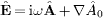and.

 Figure 1. γ-ray broadband of quasar 3C 279. MAGIC flux points from ref. , EGRET points from ref. , COMPTEL point from ref. . The solid line T + L depicts the unpolarized differential tachyon flux dNT+L/dE, obtained by adding the flux densities ρ1,2 of two ultra-relativistic electron populations, cf. table 1, and rescaled with E2 for better visibility of the spectral curvature, cf. (16). The transversal (T, dot-dashed line) and longitudinal (L, double-dot–dashed line) flux densities dNT,L/dE add up to the total unpolarized flux T + L. The exponential decay of the cascades ρ1,2 sets in at about Ecut(mt/m)kT, implying cutoffs at 180 GeV for the ρ1 cascade and 29 GeV for ρ2. The EGRET points define a spectral plateau in the MeV to GeV range typical for tachyonic cascade spectra [15, 16, 21]. The least-squares fit is done with the unpolarized tachyon flux T + L, and subsequently split into transversal and longitudinal components.

Tachyonic Poynting vectors of dipole currents

We consider a dipole current j(x, t) = pδ(x)e−iω0t + c.c., with constant dipole vector p, and use a truncated Fourier representation,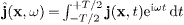, as well as the truncated delta function, to find

In this way, a well-defined meaning is given to squares of delta functions arising in the flux vectors, owing to the T → ∞ limit 2πδ2(ω;T)/T → δ(ω).

The transversal and longitudinal current transforms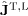defining the asymptotic outgoing wave fields are calculated via

where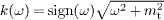is the tachyonic wave number. The projection ofonto a right-handed triad of polarization vectorsand n of the radiation field gives the transversal and longitudinal current components

Here, n = x/r is the longitudinal polarization vector, and the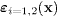define two degrees of linear transversal polarization, so thatand n constitute an orthonormal triad. The outgoing polarized field components are asymptotic solutions of the tachyonic Maxwell equations,, with amplitudes generated by the dipole current (3),

The time-averaged transversal and longitudinal Poynting vectors are assembled as 

The total transversal flux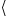STis obtained by adding the transversal polarization componentsST(i). The polarized flux components radiated by a dipole current read

The power radiated into the solid angle dΩ = sin θ dθ dis dPT,L = nST,Lr2dΩ, where we substitute the flux vectors (8) to find the power differentials of the dipole current (3),

Integration over the solid angle gives the total transversal/longitudinal power components,

which also apply for a complex dipole vector, as there are no interference terms arising in the averaged flux vectors.

The dipole approximation of a monochromatic current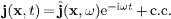is obtained by replacingby pδ(x), with. Invoking current conservation,, we write p = −iωd, with dipole moment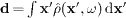, and substitute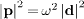in (10). Regarding the dipole, we consider an oscillating tachyonic charge with velocity, so that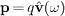, cf. before (1). The power radiated in transversal linear polarizationis found by projecting out the respective current component ofSTin (8); the squared absolute values inSTand dPT are replaced byto obtainST(i)and the linearly polarized power differentials dPT(i).

Polarized flux ratios

To derive the tachyonic Thomson cross-sections, we start with a plane wavehitting an electron carrying tachyonic charge q. The electron oscillates according to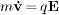. In first order in q, we may neglect the squared vector potential in the Hamilton-Jacobi equation, that is, regardas independent of the space coordinates when solving the equations of motion. We then find the Fourier amplitude of the velocity as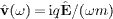. The emitted radiation stems from the current generated by the dipole; damping effects are dealt with in the following subsection.

As for the incident Fourier mode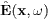, we consider polarized plane waves,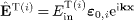or, where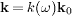,, and k0 is the unit wave vector of the incoming wave. The transversal linear polarization vectors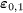andof the incident wave are chosen in a way to constitute with k0 an orthonormal triad,. The amplitudes EinT(i),L are arbitrary complex numbers. The incident flux carried by plane waves in the respective polarization is

We choose two real transversal polarization vectors,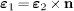and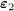as the normalized product n × k0, so that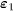lies in the plane generated by n and the incident unit wave vector k0. The scalar products of the polarization vectors of the incoming and outgoing waves are readily calculated, e.g.,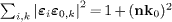. The longitudinal polarization vectors of the in- and outgoing waves are the unit wave vectors k0 and n. The angular parametrization of these products is done with polar coordinates in the coordinate frame defined by the right-handed triad,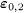and k0 of the incoming wave, so that nk0 = cos θ and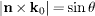.

The flux radiated into the solid angle in the respective polarization reads, cf. (8),

Here, we substitute, whereis the velocity of the electron oscillating in the incident wave. Dividing the scattered flux by the incident flux (11), we find the differential cross-sections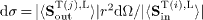.

Tachyonic Thomson cross-sections

We consider the dipole approximation of the current transform (4),, in conjunction with a damped oscillator model for the electron: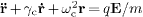, whereand, driven by a plane wave, with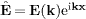. ωc is the characteristic frequency of the oscillator, and γc the damping constant; the case γc = ωc = 0 has been discussed above. In dipole approximation, we may neglect the spatial dependence of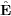, to find 

with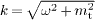. The angular parametrization is performed by means of the polarization vectors specified after (11). The incoming wave is linearly polarized,or E(k) = k0Ein in the case of longitudinal polarization.

We label the cross-sections with the polarization of the incident and outgoing flux, cf. after (12). There are four cases to distinguish. First, a transversal incoming waveand transversal outgoing radiation as defined bySoutT(j). Second, a longitudinal incoming wave E(k) = k0Ein and transversal outgoing radiation, implyingSoutT(2)(k0)= 0 since. The third combination is a transversal incoming wave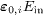and longitudinally polarized outgoing radiation, and the fourth cross-section refers to longitudinal in- and outgoing modes:

Here, the incident transversal radiation is a polarization average, and a summation is performed over the outgoing linear transversal polarizations in dσT→T. The transversal fraction of the scattered longitudinal radiation is linearly polarized, as dσL→T(2) = 0.

Polarization of tachyonic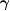-ray flares: The case of quasar 3C 279

Figures 1 and 2 show the tachyonic cascade fit of the active galactic nucleus 3C 279 . The cascades are plots of the E2-rescaled flux densities

where d is the distance to the source, and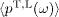the transversal/longitudinal tachyonic spectral density averaged over a thermal ultra-relativistic electron distribution, β = m/(kT) . The least-squares fit is performed with the total unpolarized flux density dNT+L = dNT + dNL. The cascades are labeled ρ1,2 in the figures, and the thermodynamic parameters of the electron populations generating them are listed in table 1. The details of the spectral fitting have been explained in refs. [6, 16]. The electron count is calculated as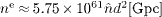, where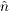defines the tachyonic flux amplitude extracted from the fit. The cutoff parameter is related to the electron temperature by kT[TeV]5.11 × 10−7/β, and the internal energy estimates of the source populations in table 1 are based on U[erg] ~ 2.46 × 10−6ne/β.

 Figure 2. Close-up of the MAGIC spectrum in fig. 1. T and L stand for the transversal and longitudinal flux components, and T + L labels the unpolarized flux. Comparing to the γ-ray blazars in ref.  at much lower redshift, or to the Galactic γ-ray binaries in refs. [13, 15], there is no indication of absorption in the spectral slopes. The spectral curvature of the Galactic sources is even more pronounced than of quasar 3C 279 at z0.538. The shape of the rescaled flux density E2 dNT+L/dE is intrinsic, generated by the Boltzmann factor of the thermal electron densities rather than by intergalactic attenuation.

 Table 1. Electronic source distributions ρi generating the tachyonic cascade spectrum of quasar 3C 279. ρ1,2 denote thermal ultra-relativistic Maxwell-Boltzmann densities with cutoff parameter β in the Boltzmann factor.determines the amplitude of the tachyon flux generated by the electron density ρi, from which the electron count ne is inferred at a distance of 2.4 Gpc, cf. after (16). kT is the temperature and U the internal energy of the electron plasma. Each cascade depends on two fitting parameters β and, extracted from the χ2-fit T + L in fig. 1.
 3C 279 βne kT(TeV) U(erg) ρ1 1.16 × 10−8 1.5 × 10−3 4.8 × 1059 44 1.0 × 1062 ρ2 7.41 × 10−8 2.5 × 10−2 8.1 × 1060 6.9 2.7 × 1062

The redshift z0.538 of quasar 3C 279  translates into a distance of 2.4 Gpc via d[Gpc]4.4z, with h00.68. High-energy γ-ray spectra of blazars are usually assumed to be generated by inverse Compton scattering or pp scattering followed by π0 decay . Both mechanisms result in a flux of GeV-TeV photons partially absorbed by interaction with infrared background photons. By contrast, there is no absorption of tachyonic γ-rays, since tachyons cannot directly interact with photons. The spectral curvature apparent in double-logarithmic plots of the E2-rescaled flux densities (16) is intrinsic, caused by the Boltzmann factor of the thermal electron plasma generating the tachyon flux. The curvature in the γ-ray flare spectra of active galactic nuclei does not increase with distance. To see this, we may compare figs. 1 and 2 to the spectral maps of the BL Lacertae objects H1426 + 428 (z0.129, 570 Mpc) and 1ES 1959 + 650 (z0.047, 210 Mpc) in ref. , or to the blazars 1ES 0229 + 200 (z0.140, 620 Mpc) and 1ES 0347 − 121 (z0.188, 830 Mpc) in ref. . There is no correlation between distance and spectral curvature visible. The common feature in the γ-ray wideband of these blazars is an extended spectral plateau in the MeV-GeV region.

Conclusion

We studied the polarization of tachyon radiation, in particular the effect of polarization on the scattering of tachyons by electrons. We calculated the polarized Thomson cross-sections and showed that longitudinal radiation is fractionally converted into transversal radiation and vice versa in this scattering process. Another way to determine the polarization of tachyons is provided by ionization cross-sections , which also peak at different scattering angles for transversal and longitudinal tachyons like the differential cross-sections in (15). Two γ-ray flares of quasar 3C 279, the most distant high-energy γ-ray blazar detected so far, were fitted with a tachyonic cascade spectrum, and the longitudinal and transversal radiation components were extracted from the unpolarized fit. Table 1 contains estimates of the thermodynamic parameters of the ultra-relativistic electron plasma generating the superluminal cascades. The EGRET and COMPTEL flux points define a spectral plateau extending over the MeV range to low GeV energies and terminating in exponential decay. This plateau as well as the curved spectral slope defined by the MAGIC points in fig. 2 are reproduced by the cascade fit.

The γ-ray wideband in fig. 1 is to be compared to the spectral maps of the Galactic γ-ray binaries in refs. [13, 15], the binary pulsar PSR B1259 − 63 and microquasar LS 5039, whose spectral slopes are even more strongly curved than of the quasar 3C 279 at z0.538. We may also compare the spectral map of this quasar to the γ-ray wideband of the Galactic center , and conclude that the curvature of these spectra is uncorrelated with distance. Therefore, absorption of electromagnetic radiation due to interaction with infrared background photons is not an attractive explanation of spectral curvature, since the curvature would increase with distance if affected by intergalactic absorption. There is no attenuation of the extragalactic tachyon flux, as tachyonic γ-rays cannot interact with photons.

Acknowledgments

The author acknowledges the support of the Japan Society for the Promotion of Science. The hospitality and stimulating atmosphere of the Centre for Nonlinear Dynamics, Bharathidasan University, Trichy, and the Institute of Mathematical Sciences, Chennai, are likewise gratefully acknowledged.

References


Nandikotkur G. et al 2007 Astrophys. J. 657 706

Kniffen D. A. et al 1993 Astrophys. J. 411 133

Williams O. R. et al 1995 Astron. Astrophys. 298 33

Albert J. et al 2008 Science 320 1752

Tomaschitz R. 2007 Ann. Phys. (N.Y.) 322 677

Tomaschitz R. 2008 Phys. Lett. A 372 4344

Tomaschitz R. 2000 Eur. Phys. J. B 17 523

Tanaka S. 1960 Prog. Theor. Phys. 24 171

Feinberg G. 1970 Sci. Am. 222 69

Tomaschitz R. 2001 Class. Quantum Grav. 18 4395

Tomaschitz R. 2006 Eur. Phys. J. C 45 493

Tomaschitz R. 2007 Eur. Phys. J. C 49 815

Tomaschitz R. 2007 Phys. Lett. A 366 289

Born M. and Wolf E. 2003 Principles of Optics (Cambridge: Cambridge University Press)

Tomaschitz R. 2007 Physica A 385 558

Tomaschitz R. 2007 Astropart. Phys. 27 92

Jorstad S. G. et al 2007 Astron. J. 134 799

Böttcher M. et al 2007 Astrophys. J. 670 968